﻿ 基于医学征象和卷积神经网络的肺结节CT图像哈希检索
«上一篇文章快速检索 高级检索

 智能系统学报2017, Vol. 12Issue (6): 857-864  DOI: 10.11992/tis.2017060350

### 引用本文YANG Xiaolan, QIANG Yan, ZHAO Juanjuan, et al. Hashing retrieval for CT images of pulmonary nodules based on medical signs and convolutional neural networks[J]. CAAI Transactions on Intelligent Systems, 2017, 12(6): 857-864. DOI: 10.11992/tis.201706035.### 文章历史

1. 太原理工大学 计算机科学与技术学院，山西 太原 030024;
2. 山西省煤炭中心医院 PET/CT中心，山西 太原 030012

Hashing retrieval for CT images of pulmonary nodules based on medical signs and convolutional neural networks
YANG Xiaolan1, QIANG Yan1, ZHAO Juanjuan1, DU Xiaoping2, ZHAO Wenting1
1. College of Computer Science and Technology, Taiyuan University of Technology, Taiyuan 030024, China;
2. PET/CT Center of Shanxi Coal Central Hospital, Taiyuan 030012, China
Abstract: Existing pulmonary nodule retrieval methods have two problems; it is difficult to express the characteristics of pulmonary nodules using hand-crafted features and the generated hashing codes have poor retrieval performance. To address these issues, this paper proposes a retrieval method for pulmonary nodules in CT images based on medical signs and convolutional neural networks. We first constructed accurate hashing codes using an accurate training set based on the values of the nine signs of pulmonary nodules. We then extracted the important semantic features of pulmonary nodules using convolutional neural networks and principal components analysis. In addition, we inversely solved the hashing functions by combining the hashing codes with the accurate training set. Finally, we developed a retrieval method, based on adaptive bits, to achieve fast searching for pulmonary nodule images. Extensive experiments and evaluations on data sets show that the method has high accuracy and retrieval precision in the process of pulmonary nodule image retrieval.
Key words: pulmonary nodules    medical signs    convolutional neural networks    principal components analysis    semantic features    Hashing Function    adaptive    image retrieval

1 相关工作

2 算法描述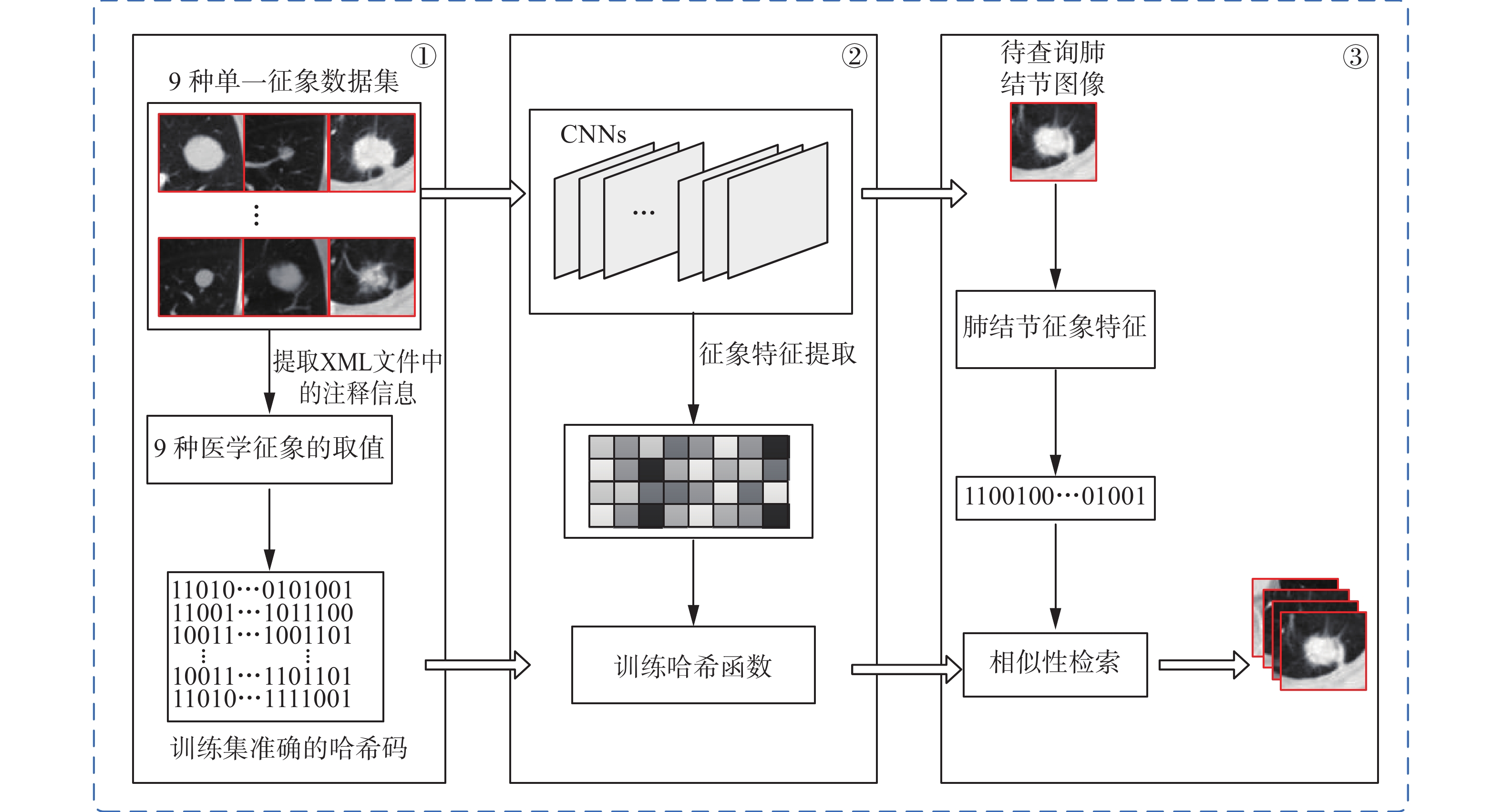图 1 基于医学征象和卷积神经网络的肺结节CT图像检索方法 Fig.1 Retrieval method of pulmonary nodules CT images based on medical signs and CNNs
2.1 构造训练集准确的二值码表 1 含有9种肺结节征象的表结构 Tab.1 The table structure that contains nine signs feature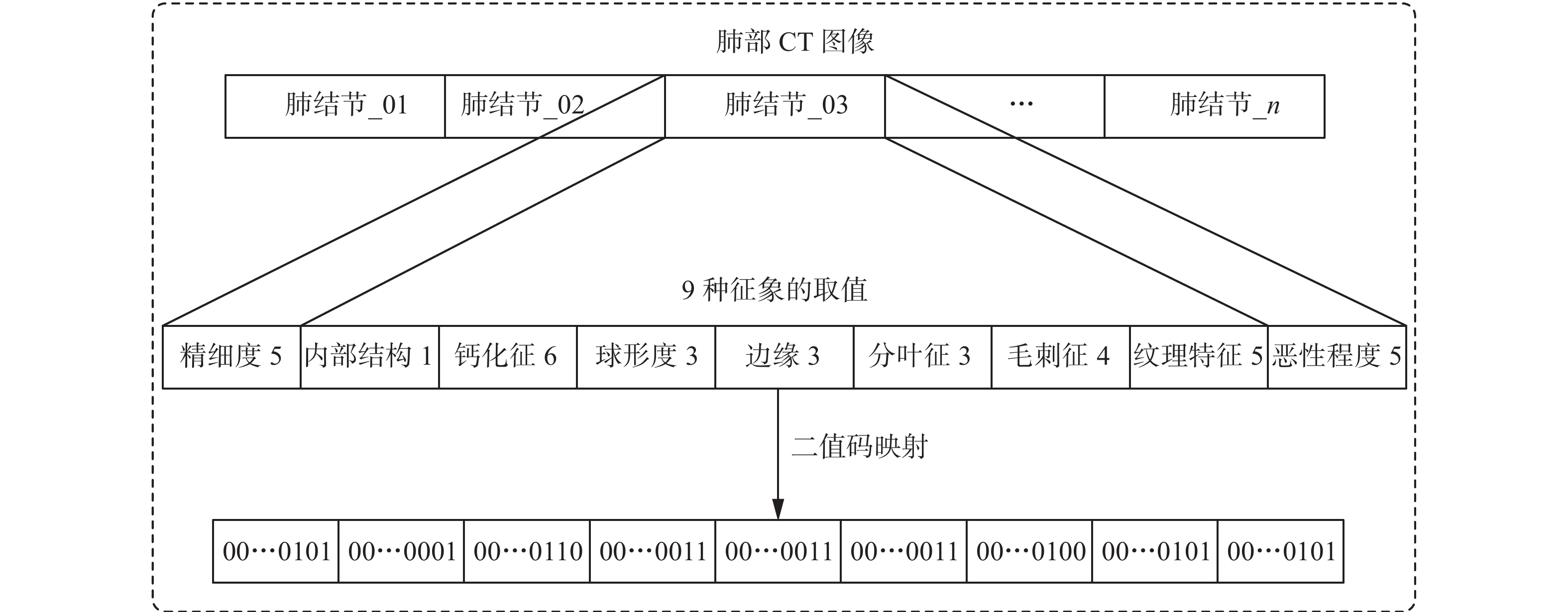图 2 肺结节征象的二值码编码 Fig.2 Binary encoding of signs of pulmonary nodules
2.2 肺结节重要语义特征提取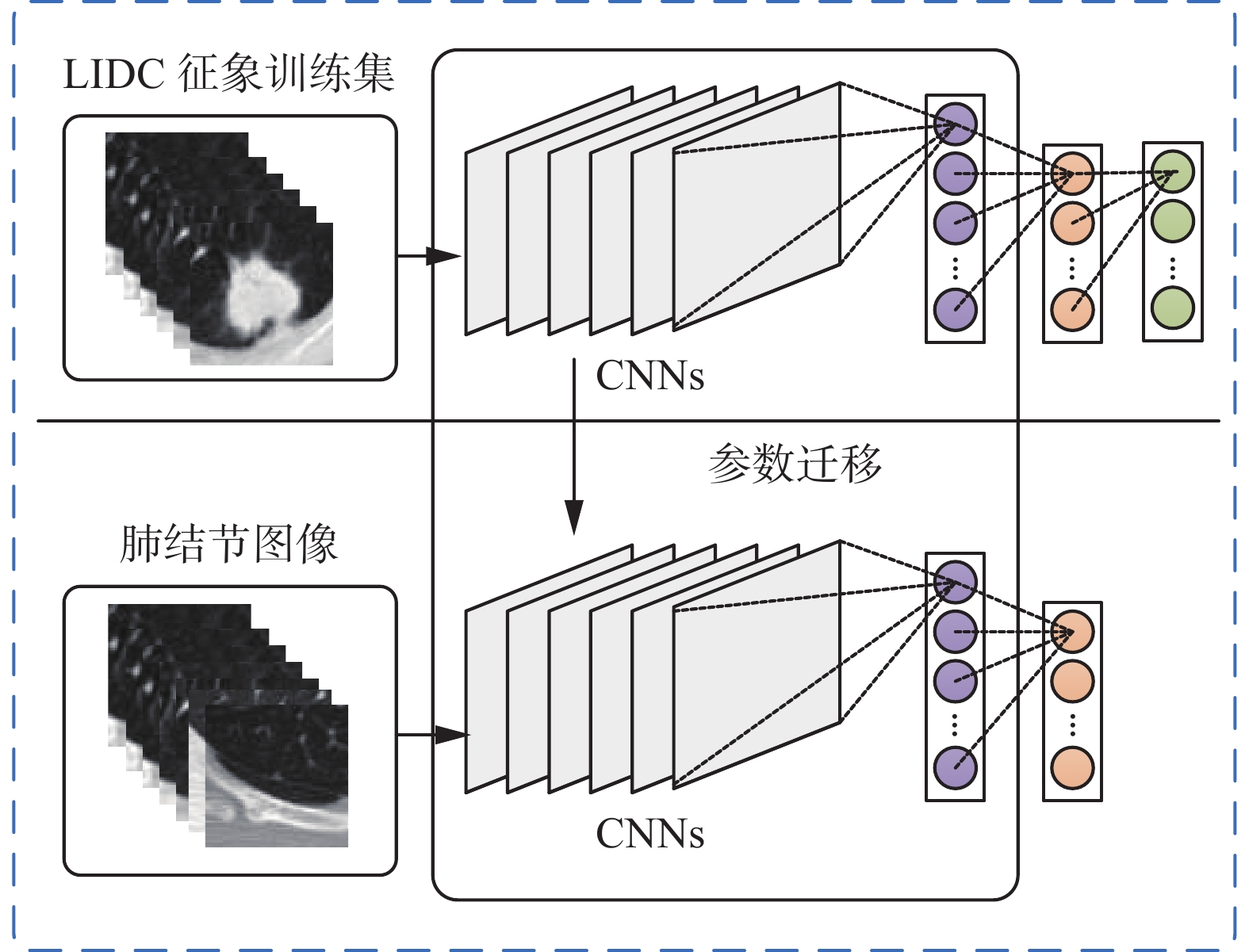图 3 基于卷积神经网络的特征提取框架 Fig.3 The convolutional neural network architecture used for features extraction

1） 将肺结节单一征象集输入到卷积神经网络中，并将网络全连接层输出的肺结节高维表示记为{(x(1), y(2), $\cdots$ , (x(n), y(n))}。

2） 通过softmax分类器建立肺结节高维表示X(i)与征象类别之间的概率分布p(y=j|x)。因为一张肺结节图像可能包含kk>1）种征象，对k种征象的可能值进行累加，同时引入权值衰减项。代价函数可表示为

 $\begin{array}{c}J(\theta ) = \displaystyle\frac{k}{n}\left[ {\sum\limits_{i = 1}^n {\sum\limits_{j = 0}^1 {1\left\{ {{y^{(i)}} = j} \right\}} } \log p\left( {{y^{(i)}} = \left. j \right|{x^{(i)}};\theta } \right)} \right] + \\\displaystyle\frac{l}{2}\sum\limits_{i = 1}^k {\sum\limits_{j = 0}^n {\theta _{ij}^2} } \end{array}$ (1)

3） 优化代价函数，选择最优的参数组合θ对CNNs网络进行调整。去掉调整好网络中的softmax分类器层，将CNNs框架中最后一层的输出作为肺结节的高维特征 ${\mathit{\boldsymbol{X}}} = {[{x_1} \. {x_2} \, \cdots \, {x_n}]^{\rm{T}}}$

4） 计算高维特征矩阵X的PCA映射矩阵 ${\mathit{\boldsymbol{Y}}} = P{\mathit{\boldsymbol{X}}}$

2.3 哈希函数的学习

 ${[{h_1} \,\, {h_2} \,\, \cdots \,\, {h_k}]^{\rm{T}}} = {[{\rm{sign}}({\mathit{\boldsymbol{Wx}}} + b)]^{\rm{T}}}$ (2)

 $h({\mathit{\boldsymbol{x}}};{\mathit{\boldsymbol{w}}}) = {\rm{sign}}({{\mathit{\boldsymbol{w}}}^{\rm{T}}}{\mathit{\boldsymbol{x}}})$ (3)

 $\mathop {\min }\limits_{\mathit{\boldsymbol{w}}} O = \sum\limits_{i = 1}^n {\sum\limits_{j = 1}^k {({\rm{sign}}({\mathit{\boldsymbol{w}}}_j^{\rm{T}}{x_i}) \oplus {y_{ij}})} }$ (4)

 ${{\mathit{\boldsymbol{h}}}_i} = {\{ - 1,1\} ^k}$ (5)
 $\sum\limits_i {{{\mathit{\boldsymbol{h}}}_i}} = 0$ (6)
 $\frac{1}{n}\sum\limits_i {{{\mathit{\boldsymbol{h}}}_i}} {\mathit{\boldsymbol{h}}}_i^{\rm{T}} = {\mathit{\boldsymbol{I}}}$ (7)

2.4 肺结节的检索过程

1）根据式（8）～（9）计算查询结节与图像库间的汉明距离，选择汉明距离最小的前ρ个图像得到肺结节候选集I

 ${D_H}({h_q},{h_i}) = \sum\limits_{i = 1}^n {({u_i} \ne {v_i})}$ (8)
 $I = \{ {x_1},{x_2},\cdots,{x_\rho }\} \leftarrow {\rm{sort}}({D_H},'{\rm{descend}}')$ (9)

2）计算待查询结节的每一位哈希码的权值 $\{ W\} = \{ {{\mathit{\boldsymbol{\varpi}}}_1}, {{\mathit{\boldsymbol{\varpi}}}_2},\cdots \! ,{{\mathit{\boldsymbol{\varpi}}} _K}\}$

初始化权值向量 ${{\mathit{\boldsymbol{\varpi}}} _k} = \{ 1,1,\cdots \! ,1\}$

for j=1：ρ

for k=1：K

判断待查询结节图像的第k位哈希码和图像库第i幅图像的哈希码是否相同

如果相同， ${{\mathit{\boldsymbol{\varpi}}} _k} = (1 + \lambda ){{\mathit{\boldsymbol{\varpi}}} _k}$

否则， ${{\mathit{\boldsymbol{\varpi}}}_k} = \lambda {{\mathit{\boldsymbol{\varpi}}} _k}$

End for

End for

3）根据式（10）计算待查询结节与候选集I之间的加权汉明距离。

 $d({h_q},{h_I}) = \sum {_{i = 1}^k(\varpi \left| {h{q_i} - h{I_i}} \right|)}$ (10)

4）选择距离最小的数据项作为最近似结节。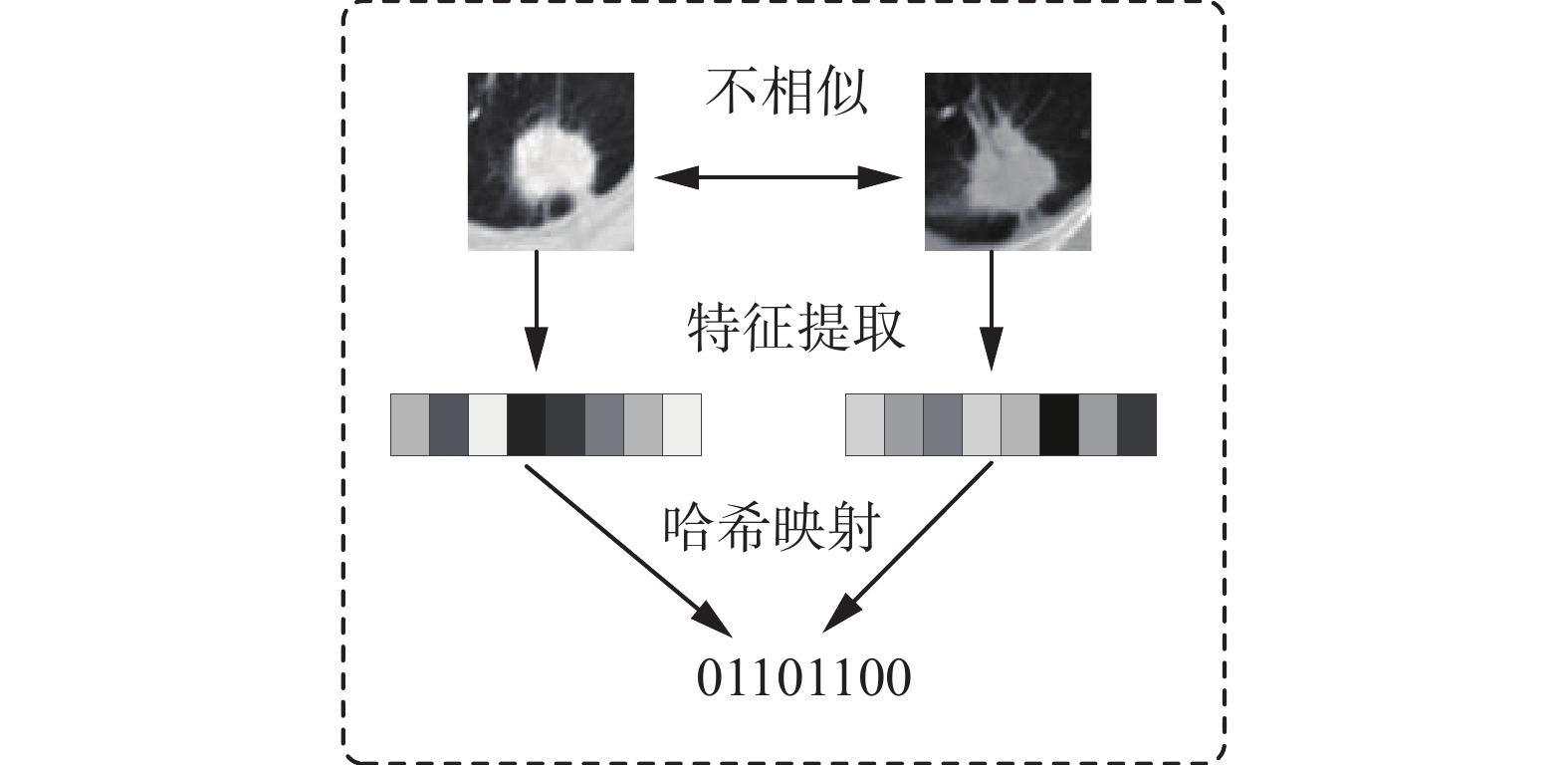图 4 不同的特征被映射成相同的哈希码 Fig.4 The phenomenon that different features are mapped to the same hashing codes
3 实验结果与分析 3.1 数据集与实验设置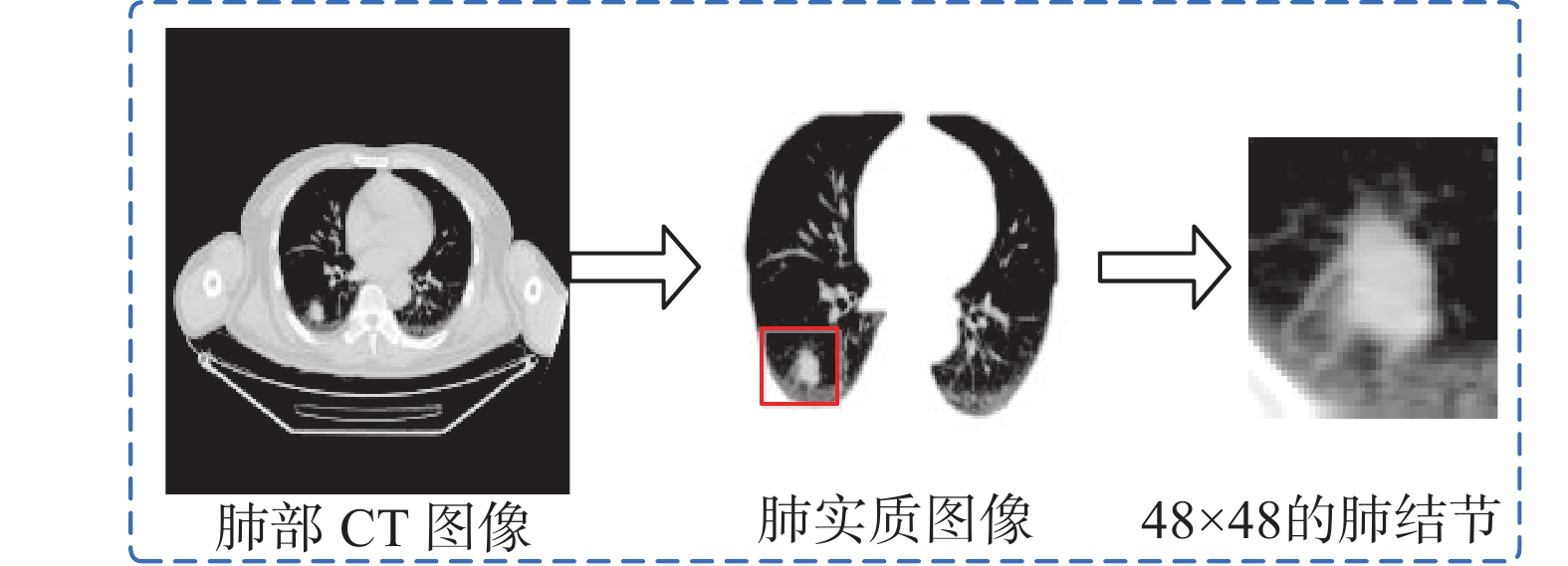图 5 肺结节的获取过程 Fig.5 Interception of pulmonary nodule

MAP反映的是在所有相似结节中的检索性能，相似结节在检索结果中的排序越靠前，MAP值就越大。P@K反映的是在检索到的前K个结节中，和查询结节相似的结节出现的概率。R@K反映的是相似结节在检索到的前K个结节中，与所有相似结节的比值。相关公式的定义为

 ${\rm{MAP}}\left( q \right) = \frac{1}{q}\sum\limits_{j = 1}^{\left| q \right|} {\frac{1}{{{m_j}}}} \sum\limits_{k = 1}^{{m_j}} {\frac{k}{{{R_{jk}}}}}$ (11)
 $P@K = \frac{{\# \left\{ {{\rm{similar}}\;{\rm{lung}}\;{\rm{nodules}}\;{\rm{images}}\;{\rm{in}}\;{\rm{top}}\;{\rm{k}}\;{\rm{results}}} \right\}}}{k}$ (12)
 $R@K = \frac{{\# \left\{ {{\rm{similar}}\;{\rm{lung}}\;{\rm{nodules}}\;{\rm{images}}\;{\rm{in}}\;{\rm{top}}\;{\rm{k}}\;{\rm{results}}} \right\}}}{{\# \left\{ {{\rm{all}}\;{\rm{similar}}\;{\rm{lung}}\;{\rm{nodules}}\;{\rm{images}}} \right\}}}$ (13)
3.2 实验结果分析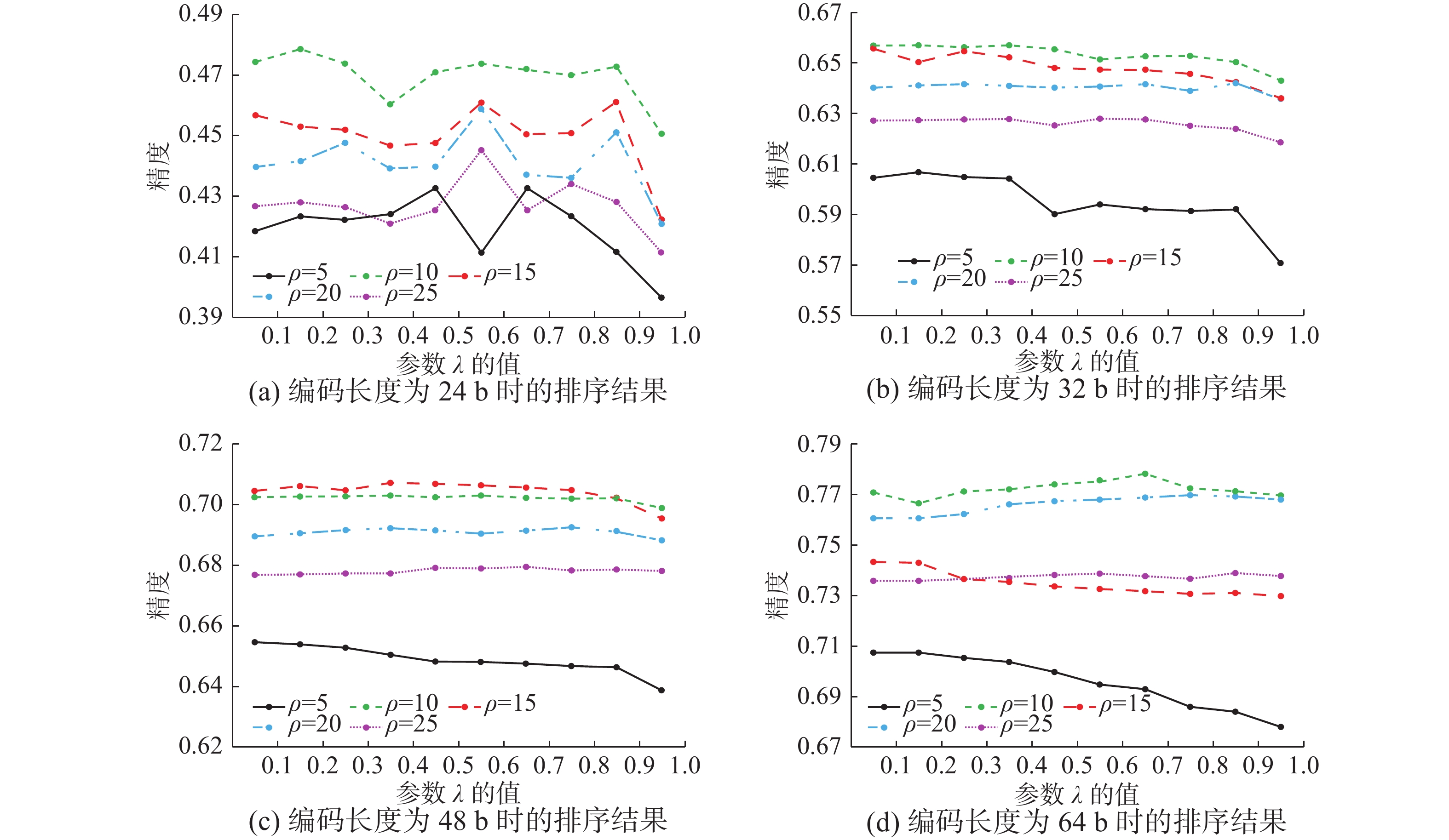图 6 本文方法在编码长度为24 b、32 b、48 b和64 b时不同λ值和ρ的排序精度 Fig.6 The sorting precision of our method with different λand ρfor different hashing bits on 24, 32, 48, and 64 bits, respectively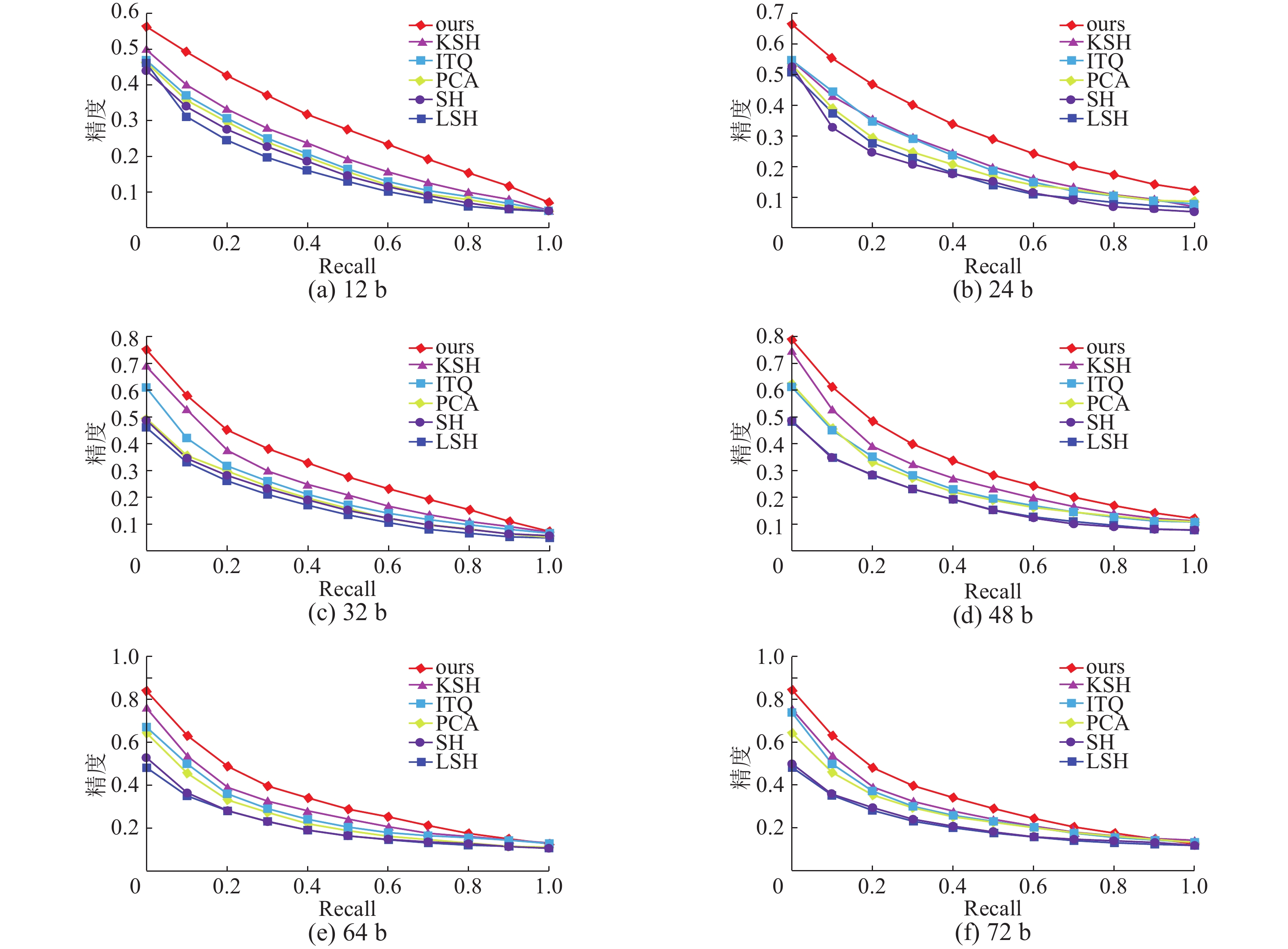图 7 不同哈希方法的查全率–查准率曲线图 Fig.7 Recall-precision curves for different hash methods表 2 不同编码长度下两种特征的MPA值 Tab.2 The two features of MAP (%) on r bits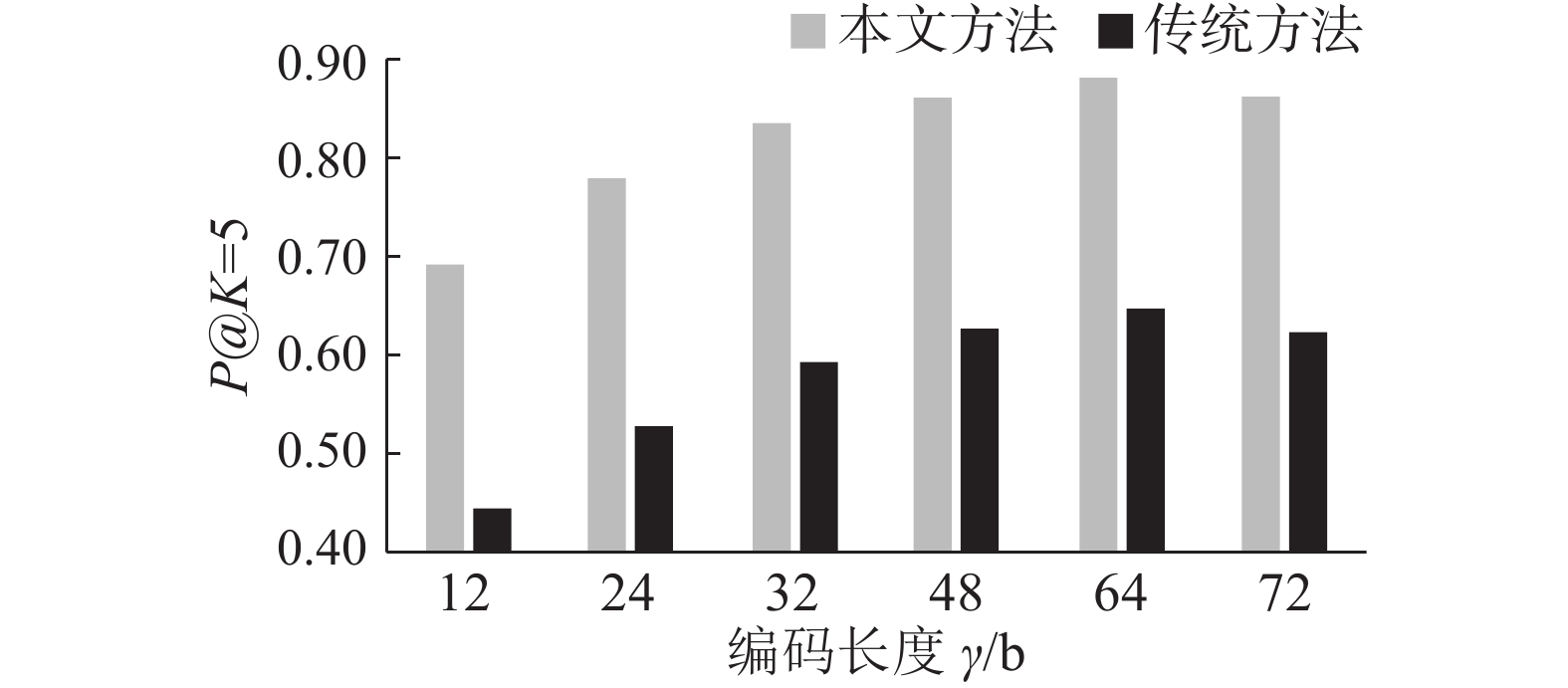图 8 不同汉明排序方法的检索结果 Fig.8 Retrieval results with different Hamming sorting methods
4 结束语

  REBECCA L, KIMBERLY D M, STACEY A F, et al. Cancer statistics[J]. CA: a cancer journal for clinicians, 2017, 67(3): 177. (0)  ZHAO Y, BOCK G H D, VLIEGENTHART R, et al. Performance of computer-aided detection of pulmonary nodules in low-dose CT: comparison with double reading by nodule volume[J]. European radiology, 2012, 22(10): 2076. (0)  CHEN S, NI D, QIN J, et al. Bridging computational features toward multiple semantic features with multi-task regression: a study of CT pulmonary nodules[M]//Medical Image Computing and Computer-Assisted Intervention-MICCAI 2016. Springer International Publishing, 2016: 53–60. (0)  VADLAMUDI L N, VADDELLA R P V, DEVARA V. Robust image hashing technique for content authentication based on DWT[C]//Proceedings of International Conference on Computer Vision and Image Processing. Singapore: Springer, 2017: 189–191. (0)  GIONIS A, IINDYK P, MOTWANI R. Similarity search in high dimensions via Hashing[C]//International Conference on Very Large Data Bases. Morgan Kaufmann Publishers Inc. 2000: 518–529. (0)  WEISS Y, TORRALBA A, FERGUS R. Spectral Hashing[J]. Proc nips, 2008, 282(3): 1753-1760. (0)  GONG Y, LAZEBNIK S, GORDO A, et al. Iterative quantization: a procrustean approach to learning binary codes for large-scale image retrieval[J]. IEEE transactions on pattern analysis and machine intelligence, 2013, 35(12): 2916-29. (0)  LIU W, WANG J, JI R, et al. Supervised hashing with kernels[C]//2012 IEEE Conference on Computer Vision and Pattern Recognition (CVPR). Providence, USA, 2012: 2074–2081. (0)  JING M, ZHANG S, HUANG J, et al. Joint kernel-based supervised hashing for scalable histopathological image analysis[C]//Medical Image Computing and Computer-Assisted Intervention 2015. Springer International Publishing, 2015, 1: 558–560. (0)  LIU J, ZHANG S, LIU W, et al. Scalable mammogram retrieval using composite anchor graph hashing with iterative quantization[J]. IEEE transactions on circuits and systems for video technology, 2016(99): 1–1. (0)  LIU H M, WANG R P, SHAN S, et al. Deep supervised hashing for fast image retrieval[C]//Proceedings of International Conference on Computer Vision and Pattern Recognition (CVPR). Las Vegas, USA, 2016: 2064–2072. (0)  YANG H F, LIN K, CHEN C S. Supervised learning of semantics-preserving hash via deep convolutional neural networks[J]. IEEE transactions on pattern analysis and machine intelligence, 2015(99): 1–1. (0)  ARMATO S, MCLENNAN G, MCNITTt-GRAY M, et al. WEB201B02: the lung image database consortium (LIDC) and image database resource initiative (IDRI): a completed public database of CT scans for lung nodule analysis[J]. Medical physics, 2010, 37(6): 3416-3417. (0)  YANG X, CHENG K T. Local difference binary for ultrafast and distinctive feature description[J]. IEEE transactions on pattern analysis and machine intelligence, 2014, 36(1): 188-94. (0)  TARANDO S R, FETITA C. Increasing CAD system efficacy for lung texture analysis using a convolutional network[C]//Proceedings of SPIE 9785, Medical Imaging. San Diego, USA, 2016: 97850Q. (0)  BABENKO, SLESAREV A, CHIGORIN A, et al. Neural codes for image retrieval[M]. Springer International Publishing, 2014: 584–599. (0)  FU H, KONG X, WANG Z. Binary code reranking method with weighted hamming distance[J]. Multimedia tools and applications, 2016, 75(3): 1391-1408. (0)  王超, 王浩, 王伟. 基于优化ROI的医学图像分割与压缩方法研究[J]. 重庆邮电大学学报: 自然科学版, 2015, 27(2): 279-284. WANG Chao, WANG Hao, WANG Wei, et al. Study of optimized ROI based medical image segmentation and compression method[J]. Journal of Chongqing university of posts and telecommunications: natural science edition, 2015, 27(2): 279-284. (0)  LIAO X, ZHAO J, CHENG J, et al. A segmentation method for lung parenchyma image sequences based on superpixels and a self-generating neural forest[J]. Plos one, 2016, 11(8): e0160556. (0)